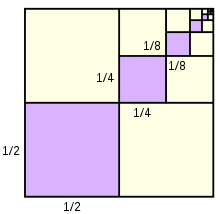# Finite geometric sequence

Find the remaining unknown characteristics in the finite geometric sequence, if given: a1 = 18, an = 13122, sn = 19674, n =?, q =?

n =  7
q =  3

### Step-by-step explanation:Did you find an error or inaccuracy? Feel free to write us. Thank you!

Tips to related online calculators
Looking for help with calculating roots of a quadratic equation?
Do you have a linear equation or system of equations and looking for its solution? Or do you have a quadratic equation?